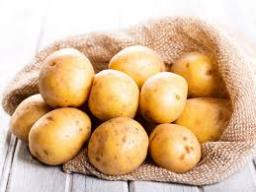The farmer

The farmer brought potatoes to the market. In the first hour he sold two-fifths of the potatoes brought in, in the second hour he sold five-sixths of the remaining potatoes, and in the third hour, he sold the last 40 kg of potatoes.

1. Express a fraction of what portion left after the first hour of sale.
2. Calculate how many kilograms of potatoes the farmer sold in the second hour.
3. Calculate how many kilograms of potatoes the farmer brought to the market.

a =  3/5
b =  200 kg
c =  400 kg

Step-by-step explanation:

2/5c + b + 40 = c
b = 5/6 (c-2/5c)

2/5•c + b + 40 = c
b = 5/6•(c-2/5•c)

5b-3c = -200
30b-15c = 0

b = 200
c = 400

Our linear equations calculator calculates it.Did you find an error or inaccuracy? Feel free to write us. Thank you!Tips to related online calculators
Need help to calculate sum, simplify or multiply fractions? Try our fraction calculator.
Do you have a linear equation or system of equations and looking for its solution? Or do you have a quadratic equation?